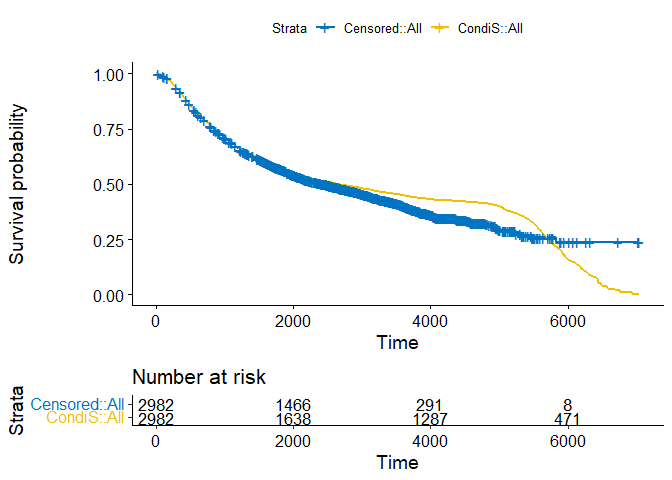# Vignette for the CondiS Package

CondiS is an R package that imputes survival time for censored observations. It allows the direct application of standard machine learning techniques for regression modeling once the imputed survival time is obtained. This vignette shows the use of CondiS package and introduce the things CondiS can do for you. CondiS was created by Yizhuo Wang, Xuelin Huang, Ziyi Li and Christopher R. Flowers, and is now maintained by Yizhuo Wang.

Install CondiS using the code below to to ensure that all the needed packages are installed.

# install.packages("CondiS", dependencies = c("survival", "caret"))

library(CondiS)

CondiS has two functions to help impute the survival times as much alike as true survival times for the censored observations. A built-in R dataset in the survival package, rotterdam, is used here to demonstrate the usages of these two functions.

## CondiS function

The imputed survival times for censored observations are generated based on their conditional survival distributions derived from the Kaplan-Meier estimator. Below are the input parameters of the CondiS function:

• time: The follow up time for right-censored data.
• status: The censoring indicator, normally 0=right censored, 1=event at time.
• tmax: A self-defined time-of-interest point; if left undefined, then it is defaulted as the maximum follow up time.
library(kernlab)
library(purrr)
library(tidyverse)
library(survival)

data(cancer, package="survival")

status <- pmax(rotterdam$recur, rotterdam$death)
rfstime <- with(rotterdam, ifelse(recur==1, rtime, dtime))
rotterdam <- rotterdam[2:11]
rotterdam$status = status rotterdam$rfstime = rfstime
fit <- survfit(Surv(rfstime, status) ~ 1, data = rotterdam)

# Obtain the imputed survival time
pred_time = CondiS(rfstime, status)
rotterdam$pred_time = pred_time rotterdam$status2 = rep(1,length(status))
fit_2 <- survfit(Surv(pred_time, status2) ~ 1, data = rotterdam)

# Visualization
library(survminer)

combined <-
list(Censored = fit,
CondiS = fit_2)

ggsurvplot(
combined,
data = rotterdam,
combine = TRUE,
censor = TRUE,
risk.table = TRUE,
palette = "jco"
)## CondiS-X function

The imputed survival times are further improved by incorporating the covariate information through machine learning modeling (CondiS-X). Below are the input parameters of the CondiS-X function:

• pred_time: The imputed follow up time for right-censored data.
• status: The censoring indicator, normally 0=right censored, 1=event at time.
• covariates: The additional patient data that is presumably associated with the survival time.
• method: Choose from 8 machine learning algorithms, including: “glm”, “ridge”, “lasso”, “gbm”, “rf”, “svm”, “knn”, “ann”; if missing, the default is set up as “glm”.
covariates = rotterdam[,1:10]

# Update the imputed survival time
pred_time_2 = CondiS_X(pred_time, status, covariates)
#>
#> Attaching package: 'caret'
#> The following object is masked from 'package:survival':
#>
#>     cluster
#> The following object is masked from 'package:purrr':
#>
#>     lift

rotterdam$pred_time_2 = pred_time_2 ## Perform regular machine learning analysis using CondiS-imputed time # Pre-process the data library(caret) preproc <- preProcess(rotterdam[,1:10], method = c('center', 'scale')) trainPreProc <- predict(preproc, rotterdam[,1:10]) train_control <- trainControl(method = "repeatedcv") # Train-test split set.seed(42) smp_size <- floor(0.75 * nrow(rotterdam)) train_ind <- sample(seq_len(nrow(rotterdam)), size = smp_size) train <- rotterdam[train_ind, ] test <- rotterdam[-train_ind, ] fit_svm = train( pred_time ~ .-status-status2-rfstime-pred_time, data = train, method = "svmRadial", trControl = train_control, na.action = na.omit ) pred_svm = predict(fit_svm, test) # Mean absolute error (MAE) calc_MAE <- function(actual,predicted) { error <- actual - predicted mean(abs(error)) } ## In the testing set: # The MAE of CondiS-imputed survival time and SVM-predicted survival time is: calc_MAE(test$pred_time,pred_svm)
#>  226.0307

# The MAE of the CondiS-X-imputed survival time and the SVM-predicted survival time is:

calc_MAE(test\$pred_time_2,pred_svm)
#>  181.4269